# AP Physics C: Mechanics : Understanding and Finding Center of Mass

## Example Questions

### Example Question #1 : Rotational Motion And Torque

Doing which of the following would allow you to find the center of mass of an object?

Recording its shape

Spinning it

Sliding it along a flat surface

Hanging it from a fixed point

Spinning it

Explanation:

Center of mass can be found by spinning an object. It will naturally spin around its center of mass, due to the concept of even distribution of mass in relation to the center of mass. Shape and mass are important factors in this property, but the most improtant factor is the mass distribution.

### Example Question #7 : Circular And Rotational Motion

If the fulcrum of a balanced scale is shifted to the left, what type of adjustment must be made to rebalance the scale?

Apply the same amount of mass to both ends

Apply more mass to the left end

If the scale was initially balanced, moving the fulcrum will not change this

Apply more mass to the right end

Apply more mass to the new position of the fulcrum

Apply more mass to the left end

Explanation:

Changing the position of the fulcrum by moving it to the left means the center of mass will be to the right of the new position. Therefore, the scale will tip right. Adding more mass to the left end will rebalance the scale. None of the other options make sense. Adding more mass to the new fulcrum position will not change the balance of the scale because that mass is a negligible distance from the new fulcrum position and does nothing to change the masses on either side.

### Example Question #8 : Circular And Rotational Motion

If two masses,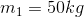and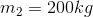are placed on a seesaw of length, where must the fulcrum be placed such that the seesaw remains level?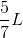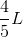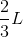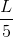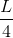Explanation:

This question asks us to find the center of mass for this system. We know that the center of mass resides a distance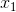from the first mass such that: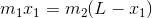In this case: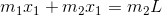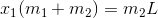Plug in known values and solve.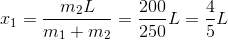### Example Question #9 : Circular And Rotational Motion

Three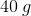point masses are at the points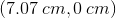,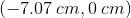and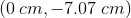and a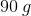point mass is at the point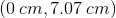.

How far from the origin is the center of mass of the system?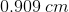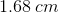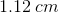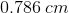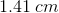Explanation:

To find the center of mass, we have to take the weighted average of the x coordinates and the y coordinates.Measures:MeasuresFirst, we take the weighted measurement of the x-axis: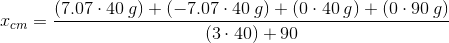We can see that the result of the x-axis contribution is equal to.

Now, let's look at the y-axis contribution: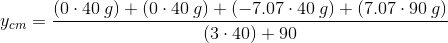This equals toNow that we have the x and y components, we take the root of squares to get the final answer: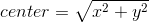This will give us